k-means

K-Means Clustering AlgorithmDefinition:

This nonheirarchial method initially takes the number of components of the population equal to the final required number of clusters. In this step itself the final required number of clusters is chosen such that the points are mutually farthest apart. Next, it examines each component in the population and assigns it to one of the clusters depending on the minimum distance. The centroid's position is recalculated everytime a component is added to the cluster and this continues until all the components are grouped into the final required number of clusters.Example:
Let us apply the k-Means clustering algorithm to the same example as in the previous page and obtain four clusters :
Food item # Protein content, P Fat content, F
Food item #1 1.1 60
Food item #2 8.2 20
Food item #3 4.2 35
Food item #4 1.5 21
Food item #5 7.6 15
Food item #6 2.0 55
Food item #7 3.9 39

Let us plot these points so that we can have better understanding of the problem. Also, we can select the three points which are farthest apart.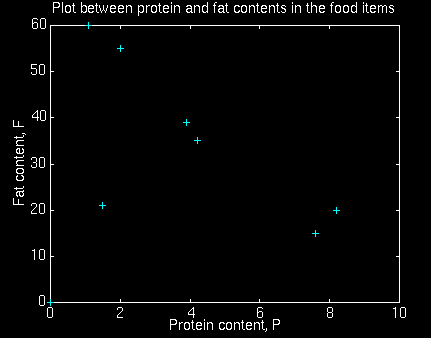We see from the graph that the distance between the points 1 and 2, 1 and 3, 1 and 4, 1 and 5, 2 and 3, 2 and 4, 3 and 4 is maximum.
Thus, the four clusters chosen are :
Cluster number Protein content, P Fat content, F
C1 1.1 60
C2 8.2 20
C3 4.2 35
C4 1.5 21

Also, we observe that point 1 is close to point 6. So, both can be taken as one cluster. The resulting cluster is called C16 cluster. The value of P for C16 centroid is (1.1 + 2.0)/2 = 1.55 and F for C16 centroid is (60 + 55)/2 = 57.50.
Upon closer observation, the point 2 can be merged with the C5 cluster. The resulting cluster is called C25 cluster. The values of P for C25 centroid is (8.2 + 7.6)/2 = 7.9 and F for C25 centroid is (20 + 15)/2 = 17.50
The point 3 is close to point 7. They can be merged into C37 cluster. The values of P for C37 centroid is (4.2 + 3.9)/2 = 4.05 and F for C37 centroid is (35 + 39)/2 = 37.
The point 4 is not close to any point. So, it is assigned to cluster number 4 i.e., C4 with the value of P for C4 centroid as 1.5 and F for C4 centroid is 21.

Finally, four clusters with three centroids have been obtained.
Cluster number Protein content, P Fat content, F
C16 1.55 57.50
C25 7.9 17.5
C37 4.05 37
C4 1.5 21

In the above example it was quite easy to estimate the distance between the points. In cases in which it is more difficult to estimate the distance, one has to use euclidean metric to measure the distance between two points to assign a point to a cluster.
Instead of having a constant learning rate, we can also decrease it over time. A particularly interesting way of doing so is to have a separate learning rate for each unitand to set it according to the harmonic series: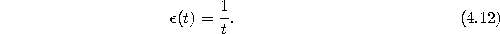Thereby, the time parameter t stands for the number of input signals for which this particular unit has been winner so far. This algorithm is known as k-means (MacQueen, 1967), which is a rather appropriate name, because each reference vector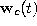is always the exact arithmetic mean of the input signals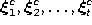it has been winner for so far. The sequence of successive values of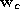is the following: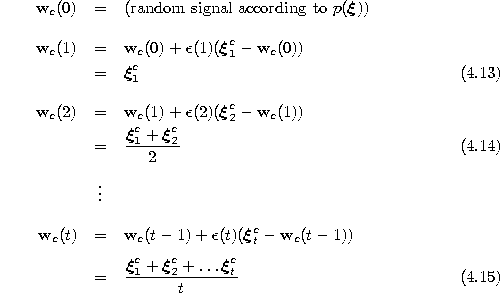One should note that the set of signals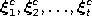for which a particular unit c has been winner may contain elements which lie outside the current Voronoi region of c. The reason is that each adaptation ofchanges the borders of the Voronoi region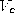. Therefore, althoughrepresents the arithmetic mean of the signals it has been winner for, at time t some of these signal may well lie in Voronoi regions belonging to other units.
Another important point about k-means is, that there is no strict convergence (as is present e.g. in LBG), the reason being that the sum of the harmonic series diverges: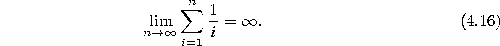Because of this divergence, even after a large number of input signals and correspondingly low values of the learning rate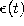arbitrarily large modifications of each input vector may occur in principal. Such large modification, however, are very improbable and in simulations where the signal distribution is stationary the reference vectors usually rather quickly take on values which are not much changed in the following. In fact, it has been shown that k-means does converge asymptotically to a configuration where each reference vectoris positioned such that it coincides with the expectation value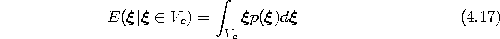of its Voronoi region(MacQueen, 1965). One can note that (4.17) is the continuous variant of the centroid condition (4.2). Figure 4.5 shows some stages of a simulation for a simple ring-shaped data distribution. Figure 4.6 displays the final results after 40000 adaptation steps for three other distribution.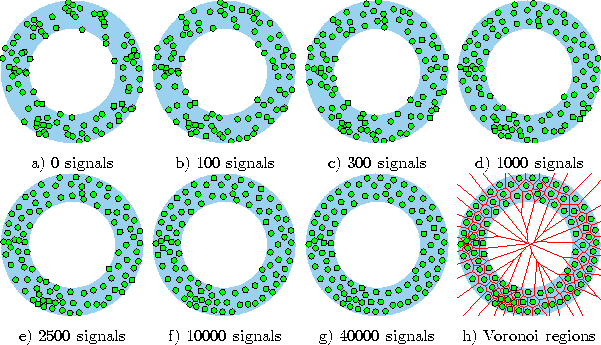Figure 4.5:   k-means simulation sequence for a ring-shaped uniform probability distribution. a) Initial state. b-f) Intermediate states. g) Final state. h) Voronoi tessellation corresponding to the final state. The final distribtion of the reference vectors still reflects the clusters present in the initial state (see in particular the region of higher vector density at the lower left).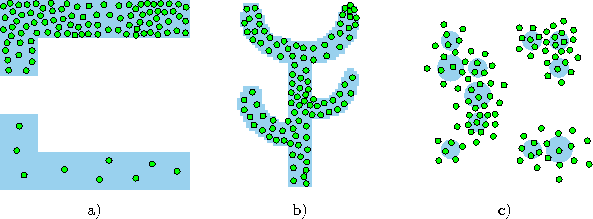Figure:   k-means simulation results after 40000 input signals for three different probability distributions (described in the caption of figure ).

Let us assume we have a classification problem, but the different classes are not known to us. We would like to separate our data into different classes according to the proximity of the samples.
For example, we have a group of patients all  suffering from the same disease and would like to see if we can find different groups with common attributes within the patients, becuase this might indicate different causes to the illness.

K-Means-Clustering - the algorithm:

1.    Select k different means at random (these will be the means of the k clusters).
2.    For each sample calculate to which of the k cluster means it is closest, and
mark it as belonging to that cluster.
3.    For each cluster calculate its new mean, using the samples marked as belonging to it.
4.    Repeat 2-3 until the new means which are calculated in 3 are close enough to the old means.
Some changes we added to the algorithm:

In our project we added a few stages to the algorithm in order to increase it's performance:

•  We start by separating the data into k log(k) clusters, and we use the same algorithm to merge those clusters into k clusters, thus enabling a greater flexibility at the beginning of the algorithm. This helps prevent false close clusters to take the place of remote clusters.

• Whenever we discover that a cluster has too few points we relocate its center to a randomly selected sample.

Our program expects a samples' matrix, and an expected number of clusters - k,
and returns a matrix that contains the noramlized distance  and related mean of each of the samples, in addition, for each cluster it returns it's center attributes, and it's estimated covariance matrix.
program files:
Example:
• Randomly create 5 clusters and then use the algorithm on it
• Example

K-Means Clustering

The algorithm essentially performs unsupervised classification on an image. That is, it does not use any apriori knowledge of the image such as the number of, and spatial relationship of, objects it may contain. The algorithm takes, as input, a set of 3-dimensional pixels, X, a set number of target clusters, k, and their corresponding initial cluster means, M1(0), M2(0), ..., Mk(0) which are also 3-dimensional pixels. As output, the algorithm produces a set of k cluster means, M1, M2, ..., Mk and a paritioning of X into k clusters, C1, C2, ..., Ck. Here is the outline of the algorithm:
1. Choose the initial cluster means, M1(0), M2(0), ..., Mk(0)
2. Partition the image pixels, X, into the k clusters
3. Recompute the cluster means, in the i th iteration, M1(i), M2(i), ..., Mk(i)
4. For each pixel in the image X, re-classify it according to the nearest cluster. The K-means clustering algorithm is also known as nearest mean reclassification algorithm for this reason.
5. If the number of pixels re-classified in step (4) falls below a threshold, then return to step (2).

The re-classification in step (4) is based on Euclidean distance. For example, the distance, d, between a pixel, Xj, and a cluster mean, Ml is given by:
d = sqrt( (Ml - Xj)T (Ml - Xj) )

Using this distance measure, k-means will partition the image into piece-wise linear boundaries. Fredrik Lidberg and Emma Lindquist describe more complex boundaries in their Clustering of Image Data 7. Moreover, they investigate the adaptive merging and splitting of clusters.
K-means essentially performs a gradient descent with respect to the squared error:
E = 1/2 ( sum-over-C1-of(X-M1)2 + sum-over-C2-of(X-M2)2 + ... + sum-over-Ck-of(X-Mk)2 )

K-means is also sensitive to the initial setting of the cluster means. Thus, although, k-means will find a local mimima, it is not guaranteed to find the global minimum (smallest error in the clustering). Morever, convergence for gradient descent can be painfully slow. Other methods methods, such as conjugate gradient 12 may prove to converge faster.
The following initial cluster means selection methods were investigated:
 Method Description Implementation Random The initial cluster means are selected at random RGB and CIE-Lab Uniform Linear The initial cluster means are selected uniformly along the line defined by [0 0 0] and [1 1 1]. RGB only Uniform Cube The initial cluster means are selected uniformily throughout the the RGB cube defined by [0 0 0] and [1 1 1]. This only works for segmentations where the cube root of the number of clusters is is a positive integer greater than one. There is a special exception for the case when number of clusters is nine. RGB only Statistical The initial cluster means are selected uniformly along the line defined by mean of the samples minus one standard deviation and the mean of the samples plus one standard deviation. RGB and CIE-Lab

CIE-Lab Color Space

CIE-Lab is first introduced by the Commission Internationale de l'Eclairage (CIE) in 1976 to solve the problem in XYZ color system, where the colorimetric distances between the individual colors don't correspond to perceived color differences.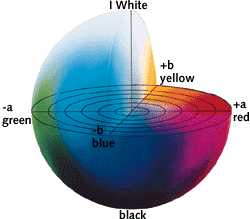This image is originally from the CIELAB Color Space web page by LinoColor
As being illustrated above, there are three components defined in the CIE-Lab color space. "L" represents the brightness, which is the axis goes from the bottom(black) to the top(white). "a" represents the hue while "b" represents the chroma.
The CIE-Lab color space has many advantages over the RGB color space. Above all, it is not device dependent therefore we can set colors as we perceive them. CIE-Lab color space is able to represent all real color gamuts as subsets.
In this project, we tried to do the color segmentation in both RGB and CIE-Lab color space. In order to perform the color segmentation in CIE-Lab color space, first it is necessary to translate the color from RGB space, which is the original image color space, to XYZ color space. The following formula can be used to achieve this: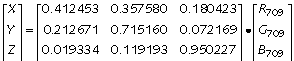To tranlate the color from XYZ color space to CIE-Lab color space, use the following formula: L = 116 Y1/3 - 16
a = 500 (X1/3 - Y1/3)
b = 200 (Y1/3 - Z1/3)

Then, we apply the k-means clustering algorithm on the colors in CIE-Lab space. The final step is to translate the segmentation result from CIE-Lab color space back to RGB color space.
Terminology:
hue: An important factor in color perception. According to the CIE, hue is the attribute of a visual sensation according to which an area appears to be similar to one of the perceived colors, red, yellow, green and bue, or a combination of two of them.
chroma: Chroma is also called saturation. Saturation refers to how far color is from a gray of equal intensity.
brightness : Brightness refers to the perceived intensity of a self-luminous object.

Region Segmentation of RGB and CIE-Lab Images With K-Means Clustering

Peter Lorenzen and Song Yao

Overview:
Region segmentation is a method that can facilitate edge detection in that it can reduce micro-edges and provide connected edges. Although many sophisticated algorithms exist for such segmentation, only the k-means clustering will be investigated for this purpose. A comparison between the RGB model and perceptually uniform CIE-Lab model will be presented.

Proposal:
Images of natural scenes with many colors will be used as they may potentially prove challenging for the clustering algorithm. That is, they may admit many clusters with large variances. We also will synthesize images for the purposes of evaluating the performance of the segmentation with respect to the driven statistical characteristics of the image (e.g. the cluster variances). We expect this approach to perform poorly on generated images whose cluster variances are relatively large.

For the natural scenes we will compare the color segmentation in the following two ways. For the RGB color model we will perform k-means clustering on an RGB image to produce M clusters from which we will generate an M-color image. For the CIE-Lab color model we will convert an RGB image to CIE-Lab color space where we will perform k-means clustering to produce M clusters in that space. From this, we will generate a M-color CIE-Lab image with the M clusters and their associated means. An M-color RGB image will then be produced by the appropriate conversion.

Our synthetic images will involve well defined topologies of regions that are uniformly colored. From these we will generate a series of synthetic images that differ, in slight color disturbances, in each of the defined regions. We will compare the results of the clustering method for these images. We are interested in how selection of variance affects the performance of the k-means clustering method.

Project Goals and Products:
We will compare the results of clustering on both color models and intend to determine, or perhaps automatically generate, reasonable values of M (the cluster cardinality). Moreover, we plan to determine a method (perhaps automatic) for selecting the initial cluster means based on image statistics as this may improve the rate of convergence for cluster mean computation.

It is our intention to provide an analysis of this technique by considering images taken from photos (and possibly the Web) as well as synthetically generated images. The report will include the various and sundry source images and their corresponding segmented images. We will also present the Matlab code used to generate the synthetic images, the segmentor, and any other salient scripts produced as a result of this effort.

Schedule:
• 04 February 1999 - Project Proposal Due This Document.
• 09 February 1999 - Phase One: Have basic functionality completed for both color models.
• 16 February 1999 - Phase Two: Have basic statistical components completed.
• 23 February 1999 - Phase Three: Have synthetic image generators completed.
• 02 March 1999 - Project Review Due: At this stage, we plan to have all the Matlab code for the segmentor and that used for performing statistical analysis completed. Essentially all the code should be built, tested, and running at this point.
• 09 March 1999 - Phase Four: Have analysis done.
• 16 March 1999 - Project Final Report Due: Full analysis and reports provided (to include relevant charts, plots, discussion, et cetera).
Note: Image acquisition and review of literature will proceed concurrently with this schedule.

Acknowledgment:
The authors would like to thank Professor Carlo Tomasi for contributing the idea of investigating CIE-Lab.

Bibliography & References:
1 D. Comaniciu and P. Meer, Robust Analysis of Feature Spaces: Color Image Segmentation, Department of Electrical Engineering and Computer Engineering, Rutgers University, Piscataway, 1997.
2 F. Dellaert, Matlab source for k-means clustering, Carnegie Mellon University, Pittsburg, Pennsylvania, 19??.
3 J. Foley and A. van Dam, et al., Computer Graphics: Principles and Practice, Addison-Wesley, Reading, Massachusetts, 1990.
4 G. Fox, R. Williams, P. Messina, Segmentation via RGB Clustering, Parallel Computing Works, Chapter 17, Morgan Kaumann Publishers, 1994.
5 R. Gonzalez and R.E. Woods, Digital Image Processing, E. Woods, Addison-Wesley, Reading, Massachusetts, 1992.
6 D. Koller, Description of k-means clustering, CS221: Artificial Intelligence: Autumn 1998, Stanford University, Stanford, California, 1998.
7 LinoColor, CIELAB Color Space
8 J. Owens and K. Starbird, Analysis and Characterization of a Color Edge Detection Algorithm, CS223B: Computer Vision: Winter 1996?, Stanford University, Stanford, California, 1996.